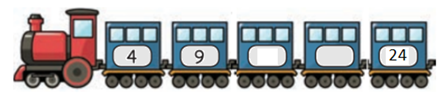Mathematics
Easy

Question

# The missing numbers in the number pattern is## 18, 22    14, 19    13, 18    14, 19Hint:

## The correct answer is: 14, 19

### The given sequence is 4, 9, … , … , 24We will subtract first term from second term.9 – 4 = 5We will try to add it to second term to see if we get complete sequence.9 + 5 = 1414 + 5 = 1919 + 5 = 24We get the last term using this rule. Therefore, we got the third and fourth terms.So, the third and fourth term will be '14, 19’.

For such questions, we should know the basic operations. When the rule used to write the pattern is not mentioned, we have to use all four operations to check the pattern. The operations are addition, subtraction, division, and multiplication.

### Related Questions to study#### With Turito Foundation.#### Get an Expert Advice From Turito.